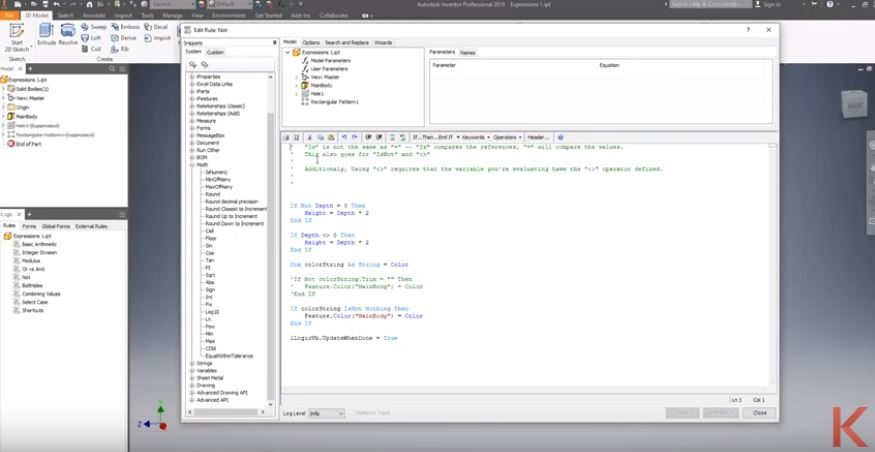# How to program in Inventor iLogic – VB.NET Operators and Expressions

When programming, it’s tough to get the results you want without knowing all the tools you have in your toolkit. Since Inventor iLogic is built on Microsoft’s VB.NET language, we can use their extensive documentation to get a better understanding of what’s possible and how we can achieve it.

We hosted an Autodesk Virtual Academy on iLogic and got a lot of requests for the links mentioned in the session. You can watch the original AVA below and keep reading to get access to all the topics and links from the session!

## Inventor iLogic- VB.NET Operators and Expressions

We’ll break this down more, but for an overview of the possible operators and expressions in Inventor iLogic, take a look here:
Microsoft’s Visual Basic Guide

Tutorials Point VB.NET Operators

### Arithmetic Operators

Microsoft’s Documentation on Arithmetic OperatorsInventor iLogic uses the following characters to do arithmetic operations like adding, subtracting, multiplying, etc.
+ (Addition) :This operator can also be used for concatenation but is not recommended
(Subtraction and Negation)
* (Multiplication)
/ or Forward Slash (Division)
\ or Backwards Slash (Integer Division)
^ (Exponentiation)
Mod Modulo operation: the remainder after division of one number by another

### Comparison Operators

Microsoft’s Documentation on Comparison Operators

Use the following characters to perform evaluations between expressions
= (Value equality):  to test if the value of the first and second expression equal to each other
<> (Value inequality): to test if the value of the first and second expression unequal to each other.
< (Less than): to test if the first value less than the second.
> (Greater than): to test if the first value greater than the second.
<= (Less than or equal to): to test if the value of the first less than or equal to the second one.
>= (Greater than or equal to) : to test if the value of the first greater than or equal to the second one.
(Is Object) reference equality
(IsNot Object) reference Inequality

### Concatenation Operators

Microsoft’s Documentation on Concatenation Operators

Two symbols can perform concatenation operations, “&” and
“+”. However, using the “+” symbol in Inventor iLogic is not recommended as it also can perform addition operations which can lead to some unexpected results.

For example, if you wanted your file name to include the height and width of your part and you used “+” to concatenate:
PartName = “MyPart”
Height = 24
Width = 13
FileName = PartName + Height + Width + “.ipt”
FileName would come out to “MyPart37.ipt” instead of the expected “MyPart2413.ipt”

### Logical Operators

Microsoft’s Documentation on Assignment Operators

Logical operators compare expressions and return a Boolean result, that is True or False.
(And): Returns True if all compared expressions evaluate to True. Will always evaluate all expressions
(Or): Returns True if at least one compared expression evaluates to True. Will always evaluate all expressions
(Xor): Returns True if exactly one compared expression evaluates to True
(AndAlso): Similar to And, will compare expressions in order and will return False immediately if an expression returns False
(OrElse): Similar to Or, will compare expressions in order and will return True as soon as one expression evaluates to True
In most scenarios, using AndAlso or OrElse is the most useful version.